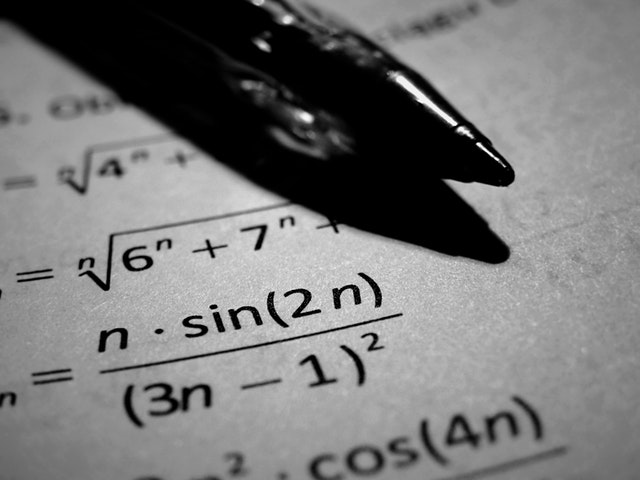# Black-ScholesIntroduction

The Black-Scholes formula is the most popular ways to calculate the true price of an option.

It is easy to calculate the intrinsic value, but the extrinsic value can be very tricky to calculate.

Black Scholes is used for calculating two types of options.

Options on stocks
Stock Options.

Fisher Black, Robert Merton and Myron Scholes originally created the Black Sholes formula in 1973.

Black Sholes uses all the ingredients that go into option pricing:

The price variation of the stock,
The time value of money,
The option’s strike price
The time to the option’s expiry.

What does the Black-Scholes Model Calculate

Securities and options that are widely traded follow a price change known as “a Geometric Brownian motion with constant shift and velocity”. Prices for options and stocks follows a normal distribution with constant standard deviations and constant growth. If you find that the Black-Scholes calculated price is greater than the current price, the formula suggests that the option should be bought and vice-versa.

Formula

The Black-Scholes formula:

C = S N(d1) – X e-rT N(d2)

where
C = price of the call option
S = price of the underlying stock
X = option exercise price
r = risk-free interest rate
T = current time until expiration
N = area under the normal curve
d1 = [ ln(S/X) + (r + σ2/2) T ] / σ T1/2
d2 = d1 – σ T1/2

σ = Standard Deviation of normally distributed stock returns

We can calculate the price of a put option with the Put-call parity:

P = Xe-rT N(-d2) – S N(-d1)

There are a few assumptions that need to be considered when calculating the price of a stock over a period period.

The Stocks price is normally distributed and so the volatility of the stock price with respect to the market is relatively constant over time.
The stock used do not pay dividends
The options can only be cashed in on expiration.
We assume that the option does not have commissions or transaction costs.
The interest on bonds and risk free rates is constant during the period.
We cannot predict market directions and markets are efficient.

Limitations of the Black-Scholes Model

The model assumes that the risk-free rate and the stock’s price volatility are constant over time. It typically misinterprets the price of options for stocks that have high-dividends.

Conclusion

The Black-Scholes model is used to calculate the mathematical value of an option. For more information and to see the weakness of this pricing model please see this Black Scholes Option Pricing Model link.

If you are ready to open a real brokerage account to start trading options, please look at this list of the best option brokers and their current offers and promotions.